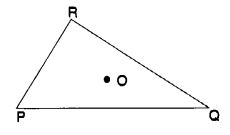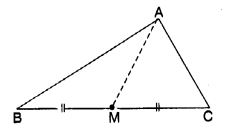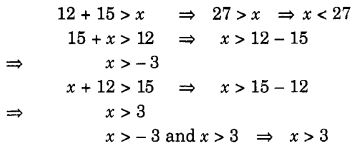NCERT Solutions for Class 7 Maths Chapter 6 The Triangle and its Properties Ex 6.4 are part of NCERT Solutions for Class 7 Maths. Here we have given NCERT Solutions for Class 7 Maths Chapter 6 The Triangle and its Properties Ex 6.4.

 Board CBSE Textbook NCERT Class Class 7 Subject Maths Chapter Chapter 6 Chapter Name The Triangle and its Properties Exercise Ex 6.4 Number of Questions Solved 6 Category NCERT Solutions

## NCERT Solutions for Class 7 Maths Chapter 6 The Triangle and its Properties Ex 6.4

Question 1.
Is it possible to have a triangle with the following sides?

1. 2 cm, 3 cm, 5 cm
2. 3 cm, 6 cm, 7 cm
3. 6 cm, 3 cm, 2 cm.

Solution:

1. Since, 2 + 3 > 5
So the given side lengths cannot form a triangle.
2. We have, 3 + 6 > 7, 3 + 7 > 6 and 6 + 7 > 3
i. e., the sum of any two sides is greater than the third side.
So, these side lengths form a triangle.
3. We have, 6 + 3 > 2, 3 + 2 $$\ngtr$$ 6
So, the given side lengths cannot form a triangle.

Question 2.
Take any point O in the interior of a triangle PQR. Is

1. OP + OQ > PQ ?
2. OQ + OR > QR?
3. OR + OP > RP ?Solution:

1. Yes ! OP + OQ > PQ …(1)
Sum of the lengths of any two sides of a triangle is greater than the length of the third side
2. Yes! OQ + OR > QR …(2)
Sum of the lengths of any two sides of a triangle is greater than the length of the third side
3. Yes! OR + OP > RP …(3)
Sum of the lengths of any two sides of a triangle is greater than the length of the third side

Question 3.
AM is a median of a triangle ABC. Is AB + BC + CA > 2 AM?
(Consider the sides of triangles ∆ ABM and ∆ AMC.)Solution:
Using triangle inequality property in triangles ABM and AMC, we have
AB + BM > AM …(1) and, AC + MC > AM …(2)
Adding (1) and (2) on both sides, we get
AB + (BM + MC) + AC > AM + AM
⇒ AB + BC + AC > 2AM

Question 4.
Is AB + BC + CD + DA > AC + BD ?Solution:
In ∆ ABC, AB + BC > AC …(1)
Sum of the lengths of any two sides of a triangle is greater than the length of the third side
In ∆ ACD, CD + DA > AC …(2)
Sum of the lengths of any two sides of a triangle is greater than the length of the third side
AB + BC + CD + DA > 2AC …(3)
In ∆ ABD, AB + DA > BD …(4)
Sum of the lengths of any two sides of a triangle is greater than the length of the third side
In ∆ BCD, BC + CD > BD …(5)
Sum of the lengths of any two sides of a triangle is greater than the length of the third side
AB + BC + CD + DA > 2BD …(6)
2 [AB + BC + CD + DA] > 2 (AC + BD)
⇒ AB + BC + CD + DA > AC + BD.

Question 5.
ABCD is a quadrilateral. Is AB + BC + CD + DA < 2 (AC + BD)?Solution:
In ∆ OAB, OA + OB > AB ….(1)
Sum of the lengths of any two sides of a triangle is greater than the length of the third side.
In ∆ OBC, OB + OC > BC ….(2)
Sum of the lengths of any two sides of a triangle la greater than the length of the third side.
In ∆ OCA,OC + OA > CA ….(3)
Sum of the lengths of any two sides of a triangle is greater than the length of the third side
Sum of the lengths of any two sides of a triangle is greater than the length of the third side
Adding (1), (2), (3) and (4),
2(OA + OB + OC + OD) > AB + BC + CD + DA
⇒ AB + BC + CD + DA < 2 (OA + OB + OC + OD)
⇒ AB + BC + CD + DA < 2(OA + OC + OB + OD)
⇒ AB + BC + CD + DA < 2 (AC + BD).

Question 6.
The lengths of the two sides of a triangle are 12 cm and 15 cm. Between what two measures should the length of the third side fall?
Solution:
Let x cm be the length of the third side.
∴ Sum of the lengths of any two sides of a triangle is greater than the length of the third side.
∴ We should have∴ The length of the third side should be any length between 3 cm and 27 cm.

We hope the NCERT Solutions for Class 7 Maths Chapter 6 The Triangle and it’s Properties Ex 6.4 help you. If you have any query regarding NCERT Solutions for Class 7 Maths Chapter 6 The Triangle and its Properties Ex 6.4, drop a comment below and we will get back to you at the earliest.